# Introduction

In this article, we will go through the tutorial for the matplotlib quiver plot for beginers. Quiver charts are generally used for physics experiments visualization. Here we will learn how to create various types of quiver plots with matplotlib with different types of examples and also learn the details that should be taken care of while working with quiver plots.

### Importing Matplotlib Library

Before beginning with this matplotlib bar plot tutorial, we’ll need the Matplotlib Library and other relevant libraries.

In :
import numpy as np
import matplotlib.pyplot as plt

### Example 1: Simple Matplotlib Quiver Plot

In the first example of the matplotlib quiver plot tutorial, we will create three different types of simple quiver charts.

The quiver function is called thrice to plot these three different plots. Each plot shows different arrow size and direction that depict different applications of this plot.

In :
X, Y = np.meshgrid(np.arange(0, 2 * np.pi, .2), np.arange(0, 2 * np.pi, .2))
U = np.cos(X)
V = np.sin(Y)

fig1, ax1 = plt.subplots()
ax1.set_title('Arrows scale with plot width, not view')
Q = ax1.quiver(X, Y, U, V, units='width')
qk = ax1.quiverkey(Q, 0.9, 0.9, 2, r'$2 \frac{m}{s}$', labelpos='E',coordinates='figure')

fig2, ax2 = plt.subplots()
ax2.set_title("pivot='mid'; every third arrow; units='inches'")
Q = ax2.quiver(X[::3, ::3], Y[::3, ::3], U[::3, ::3], V[::3, ::3],pivot='mid', units='inches')
qk = ax2.quiverkey(Q, 0.9, 0.9, 1, r'$1 \frac{m}{s}$', labelpos='E',coordinates='figure')
ax2.scatter(X[::3, ::3], Y[::3, ::3], color='r', s=5)

fig3, ax3 = plt.subplots()
ax3.set_title("pivot='tip'; scales with x view")
M = np.hypot(U, V)
Q = ax3.quiver(X, Y, U, V, M, units='x', pivot='tip', width=0.022,scale=1 / 0.15)
qk = ax3.quiverkey(Q, 0.9, 0.9, 1, r'$1 \frac{m}{s}$', labelpos='E',coordinates='figure')
ax3.scatter(X, Y, color='0.5', s=1)

plt.show()

Output: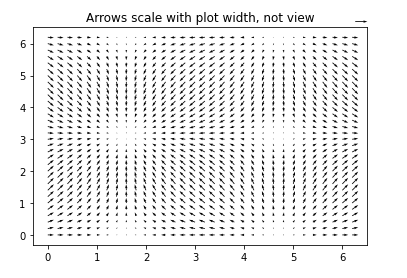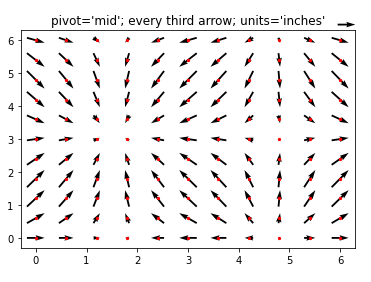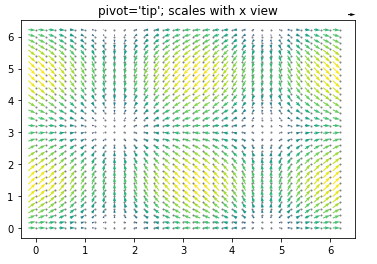### Example 2 : Gradient Based Quiver Plot

In this example, we are going to see how to build an interesting gradient-based quiver plot. This quiver plot will showcase the movement of an electrical dipole. Once we import the necessary libraries, we are required to create a function that generates the dipole potential for an electrical diploe.

Furthermore, some more functions are built that will emulate the electrical field around the diple and also help in conveying the movements.

To plot three different regions, we call the tricontour function, and lastly, the quiver function for visualizing the arrows.

In :
from matplotlib.tri import (
Triangulation, UniformTriRefiner, CubicTriInterpolator)
import matplotlib.cm as cm

#-----------------------------------------------------------------------------
# Electrical potential of a dipole
#-----------------------------------------------------------------------------
def dipole_potential(x, y):
"""The electric dipole potential V, at position *x*, *y*."""
r_sq = x**2 + y**2
theta = np.arctan2(y, x)
z = np.cos(theta)/r_sq
return (np.max(z) - z) / (np.max(z) - np.min(z))

#-----------------------------------------------------------------------------
# Creating a Triangulation
#-----------------------------------------------------------------------------
# First create the x and y coordinates of the points.
n_angles = 30

angles = np.linspace(0, 2 * np.pi, n_angles, endpoint=False)
angles = np.repeat(angles[..., np.newaxis], n_radii, axis=1)
angles[:, 1::2] += np.pi / n_angles

V = dipole_potential(x, y)

# Create the Triangulation; no triangles specified so Delaunay triangulation
# created.
triang = Triangulation(x, y)

y[triang.triangles].mean(axis=1))

#-----------------------------------------------------------------------------
# Refine data - interpolates the electrical potential V
#-----------------------------------------------------------------------------
refiner = UniformTriRefiner(triang)
tri_refi, z_test_refi = refiner.refine_field(V, subdiv=3)

#-----------------------------------------------------------------------------
# Computes the electrical field (Ex, Ey) as gradient of electrical potential
#-----------------------------------------------------------------------------
tci = CubicTriInterpolator(triang, -V)
# Gradient requested here at the mesh nodes but could be anywhere else:
E_norm = np.sqrt(Ex**2 + Ey**2)

#-----------------------------------------------------------------------------
# Plot the triangulation, the potential iso-contours and the vector field
#-----------------------------------------------------------------------------
fig, ax = plt.subplots()
ax.set_aspect('equal')
# Enforce the margins, and enlarge them to give room for the vectors.
ax.use_sticky_edges = False
ax.margins(0.07)

ax.triplot(triang, color='0.8')

levels = np.arange(0., 1., 0.01)
cmap = cm.get_cmap(name='hot', lut=None)
ax.tricontour(tri_refi, z_test_refi, levels=levels, cmap=cmap,
linewidths=[2.0, 1.0, 1.0, 1.0])
# Plots direction of the electrical vector field
ax.quiver(triang.x, triang.y, Ex/E_norm, Ey/E_norm,
units='xy', scale=10., zorder=3, color='blue',

plt.show()

Output: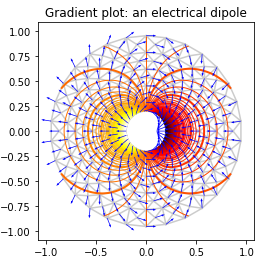### Example 3: Quiver Plot for showing Flow

These kinds of quiver plots are used in showing the movement of flow in different mediums with the help of arrows. Once the data is obtained or generated, for plotting gradient relevant values are obtained.

The quiver function is called to plot this visualization. The color of the arrows is set by using the sqrt function of numpy.

In :
x = np.arange(-2,2.2,0.2)
y = np.arange(-2,2.2,0.2)

X, Y = np.meshgrid(x, y)
z = X*np.exp(-X**2 -Y**2)

n = -2
color_array = np.sqrt(((dx-n)/2)**2 + ((dy-n)/2)**2)

fig, ax = plt.subplots(figsize=(7,7))
ax.quiver(X,Y,dx,dy,color_array)

ax.xaxis.set_ticks([])
ax.yaxis.set_ticks([])
ax.set_aspect('equal')
plt.title("Flow Based Quiver Plot")

plt.show()

Output: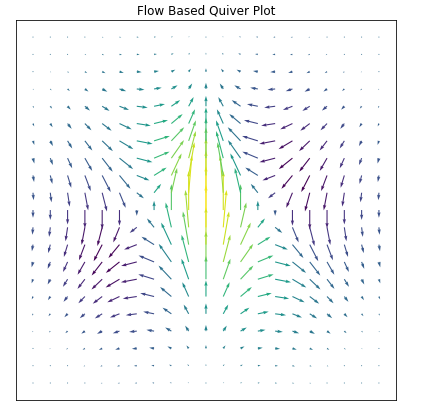### Example 4 : Stream Plot built using Quiver Plot

The last example is the stream plot depicting two point charges. With the help of streamplot we are able to plot this flow-depicting visualization.

The data for the three dimensions are passed to the streamplot function.

In :
x = np.arange(-4,4,0.2)
y = np.arange(-4,4,0.2)

X,Y = np.meshgrid(x,y)
Ex = (X + 1)/((X+1)**2 + Y**2) - (X - 1)/((X-1)**2 + Y**2)
Ey = Y/((X+1)**2 + Y**2) - Y/((X-1)**2 + Y**2)

fig, ax = plt.subplots(figsize=(6,6))

ax.streamplot(X,Y,Ex,Ey)

ax.set_aspect('equal')
ax.plot(-1,0,'-*r')
ax.plot(1,0,'-*b')
ax.set_title('Stream Plot of Two Point Charges')

plt.show()

Output: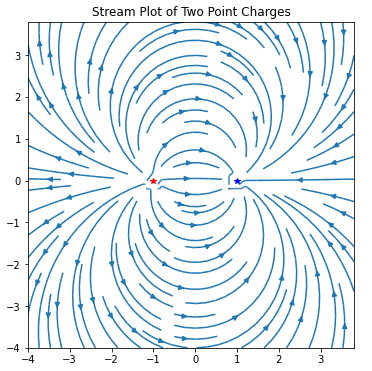## Conclusion

We have reached the end of this tutorial on matplotlib quiver plot with matplotlib. Here we learned about different types of quiver plots that can be build using matplotlib. The plots covered talk about the intricate details that should be taken care while building a variety of useful quiver plots. We also looked at how we can play around with the parameters for producing a combination of different quiver plots.

Reference –  Matplotlib Documentation